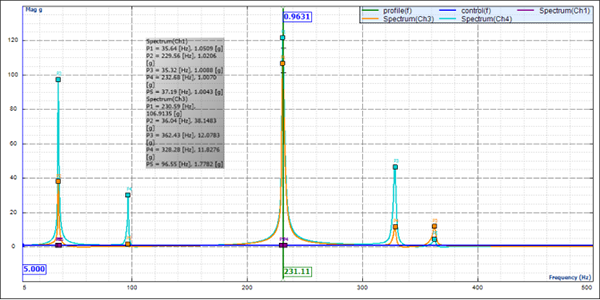# NEWS

### Sweep Frequency Vibration Test

Release time：2020-04-09　Click amount： 36
Share

The previous article introduces the random vibration test of the reliability test, and this article introduces the sweep frequency vibration test. Both the sweep frequency vibration test and random vibration test belong to the vibration test category and both use the electromagnetic vibration machine as the main test device. Different from the "random" characteristics of the random vibration test, the sweep frequency vibration test has specific frequency and magnitude at every moment. The sweep frequency vibration test is mainly used to detect the resonance points of products and determine the mechanical weakness and performance downgrade of the samples.

The sinusoidal sweep vibration test to carries out sinusoidal vibration on a sample with a given frequency range or discrete frequencies in a certain period of time. The test can check and determine the dangerous frequencies of the sample by using specified vibration response. It is a deterministic and relatively simple process, and therefore it is applicable to the diagnosis and service life test. Whether the sample is acceptable is determined also according to related specifications. In some cases, the test can be used to verify the mechanical integrity of the sample and study the dynamic characteristics.

Test spectral line: Sweep frequency vibration also presents a spectral chart. The horizontal ordinate represents frequency, and the vertical ordinate represents acceleration (displacement, speed, and so on). In the actual test, the process with the frequency changing from low to high and then to low is regarded as a scan cycle. During this period, the monitoring sensor pasted on the test sample returns the monitored value, which is compared with the input value. Then the software end automatically calculates the frequency response function (FRF), and the resonance point of the product can be clearly obtained. The vulnerable parts can be enhanced according to the resonance point.Test standards: Mainly include GB/T 2423.10 and IEC 60068-2-6.

Test classification: The test can be divide into the constant frequency vibration test and sweep frequency vibration test according to the frequency change.

Constant frequency vibration test: The frequency of sinusoidal constant frequency vibration is always unchanged. Generally, the test is to simulate the vibration caused by the rotating machinery with a fixed speed or simulate the vibration at the natural frequency of the structure. The constant frequency test contains the vibration strength test, which is to check the fatigue strength.

Frequency sweep vibration test: The frequency in the sweep frequency vibration test changes according to a certain rule. The frequency scan is divided into linear scan and logarithmic scan. In the linear scan, frequency changes linearly, that is, how many Hertz is swept per unit time, and the unit is Hz/s. The linear scan is used in the test of searching for the resonance frequency. In the logarithmic scan, frequency changes by logarithm, and the scan rate can be oct/min. The logarithmic scan indicates that the frequency octaves scanned in the same time are the same. In logarithmic scan, low frequency scan is slow while high frequency scan is fast.

Main failure modes of products

In the frame-type product structure, there are often many resonance points. If the structural strength is insufficient, the following results may occur:

Structural damage: such as fastener looseness, sheet metal cracking, and plastic shell cracking

Loose parts: such as plug-in device disconnection and keyboard slipping

Abrasion: such as cable abrasion

Electrical failure: such as IGBT explosion and communication failure

Related recommendations